# numpy.linalg.pinv¶

numpy.linalg.pinv(a, rcond=1e-15)[source]

Compute the (Moore-Penrose) pseudo-inverse of a matrix.

Calculate the generalized inverse of a matrix using its singular-value decomposition (SVD) and including all large singular values.

Parameters: a : (M, N) array_like Matrix to be pseudo-inverted. rcond : float Cutoff for small singular values. Singular values smaller (in modulus) than rcond * largest_singular_value (again, in modulus) are set to zero. B : (N, M) ndarray The pseudo-inverse of a. If a is a matrix instance, then so is B. LinAlgError If the SVD computation does not converge.

Notes

The pseudo-inverse of a matrix A, denoted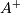, is defined as: “the matrix that ‘solves’ [the least-squares problem]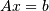,” i.e., if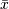is said solution, thenis that matrix such that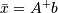.

It can be shown that if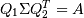is the singular value decomposition of A, then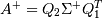, where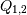are orthogonal matrices,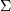is a diagonal matrix consisting of A’s so-called singular values, (followed, typically, by zeros), and then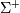is simply the diagonal matrix consisting of the reciprocals of A’s singular values (again, followed by zeros). [R47]

References

 [R47] (1, 2) G. Strang, Linear Algebra and Its Applications, 2nd Ed., Orlando, FL, Academic Press, Inc., 1980, pp. 139-142.

Examples

The following example checks that a * a+ * a == a and a+ * a * a+ == a+:

>>> a = np.random.randn(9, 6)
>>> B = np.linalg.pinv(a)
>>> np.allclose(a, np.dot(a, np.dot(B, a)))
True
>>> np.allclose(B, np.dot(B, np.dot(a, B)))
True


numpy.linalg.inv

#### Next topic

numpy.linalg.tensorinv